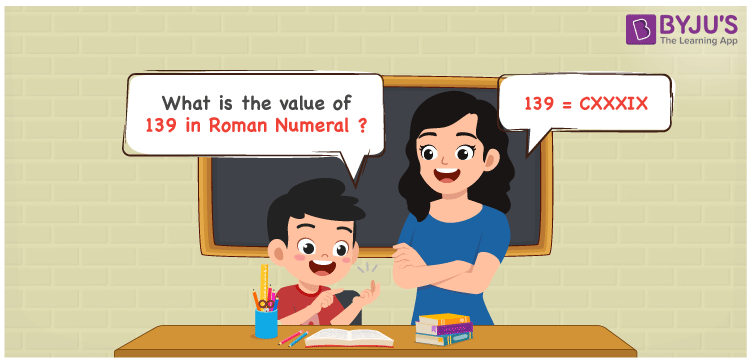# 139 in Roman Numerals

139 in Roman Numerals is CXXXIX. Any Roman numeral uses only the 7 primary symbols which are I, V, X, L, C, D, and M. Here C represents 100, X represents 10 and I represents 1. 139 can be obtained by following the addition and subtraction rule of Roman Numerals. For understanding the rules on Roman Numerals, you can refer to the link Rules to write Roman Numerals. The details on how to write 139 is given in the next section of this article.

 Number Roman Numeral 139 CXXXIX

## How to Write 139 in Roman Numerals?To convert 139 in Roman Numerals, we need to represent 139 as the sum of the fundamental symbols. 139 is nothing but 100 + 30 + 9. But neither 30 nor 1 have fundamental Roman numerals. So let’s split them again, 30 as sum of 3 tens and 9 as the difference of 10 and 1. Representing them numerically;

139 = 100 + 30 + 9

139 = 100 + (10 + 10 + 10) + (10 – 1)

139 = C + ( X + X + X) + (X – I)

139 = C + X + X + X + (IX)

139 = CXXXIX.

## Video Lesson on Roman Numerals## Frequently Asked Questions on 139 in Roman Numerals

Q1

### How to write the number 139 in Roman Numerals?

139 in Roman Numerals is written as CXXXIX.
Q2

### Does CXXXIX represent Roman Numerals?

Yes. CXXXIX represents Roman Numerals and it is the number 139
Q3

### What is the year 139 in Roman numerals?

The year 139 in Roman Numerals is CXXXIX.